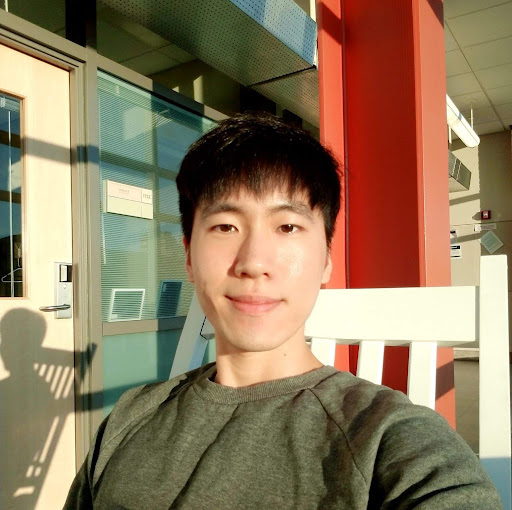Published

# Toothpaste Dispenser

A toothpaste dispenser using Texas Instruments MSP430-EXP430G2 MSP430 LaunchPad, 2 IR sensors, and a RC servo.

IntermediateShowcase (no instructions)86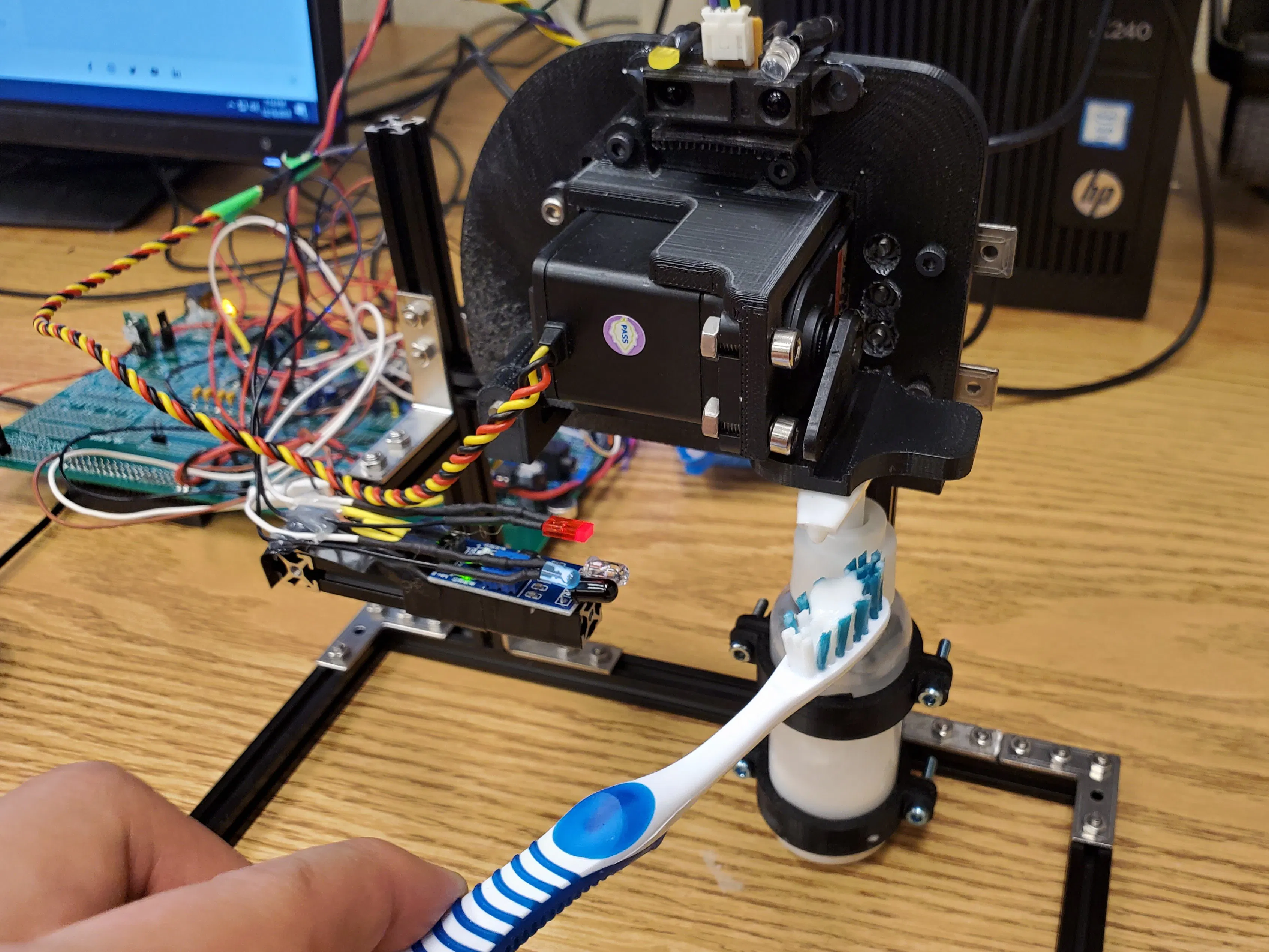## Things used in this project

### Hardware components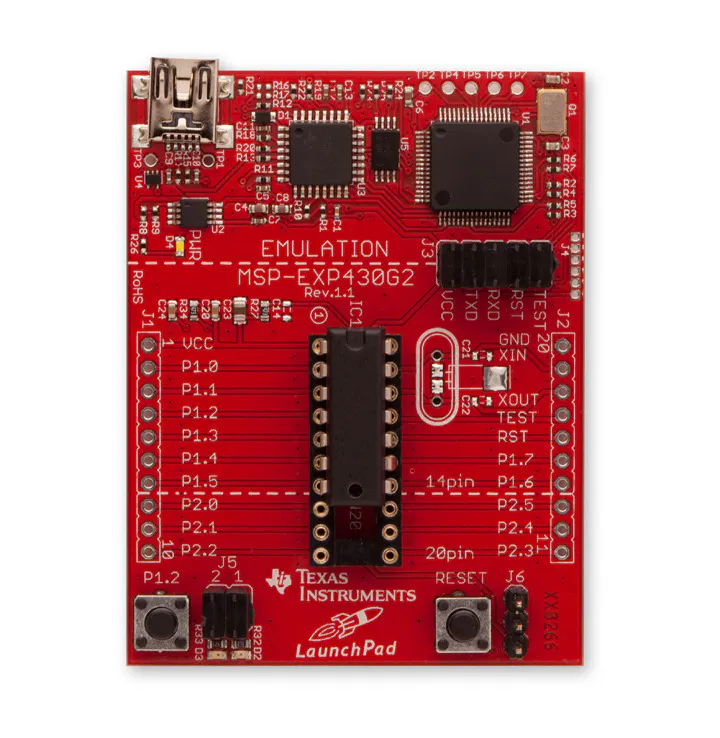×1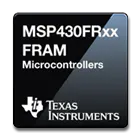Texas Instruments MSP430 Microcontroller
×1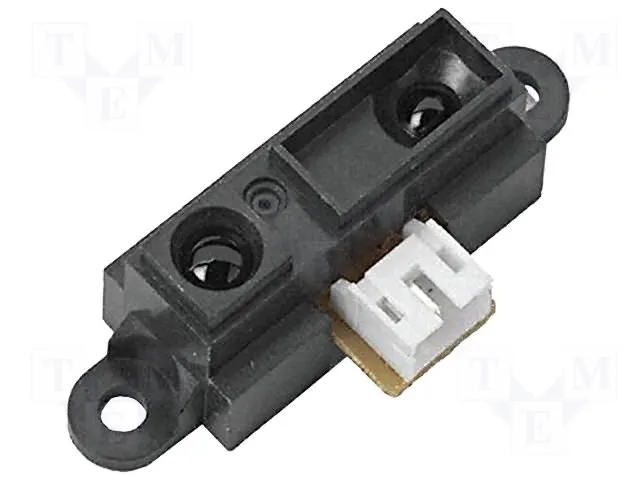Proximity Sensor
×1
 Hitec Hs-755HB
×1
 FC-51 Infrared Proximity Sensor
×1

### Software apps and online servicesTexas Instruments Code Composer Studio

Solidworks Files

## Schematics

### Schematic1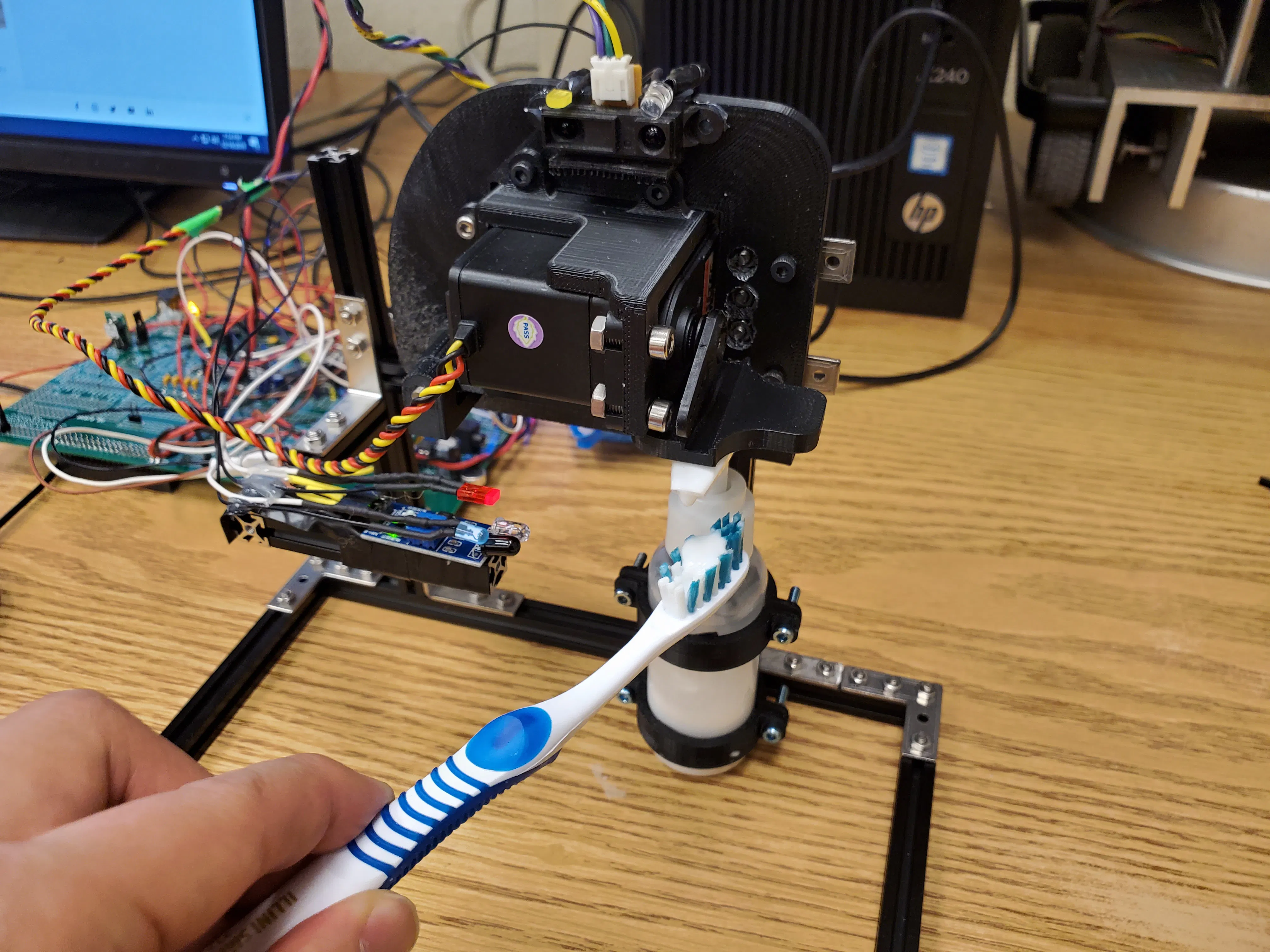### Schematic2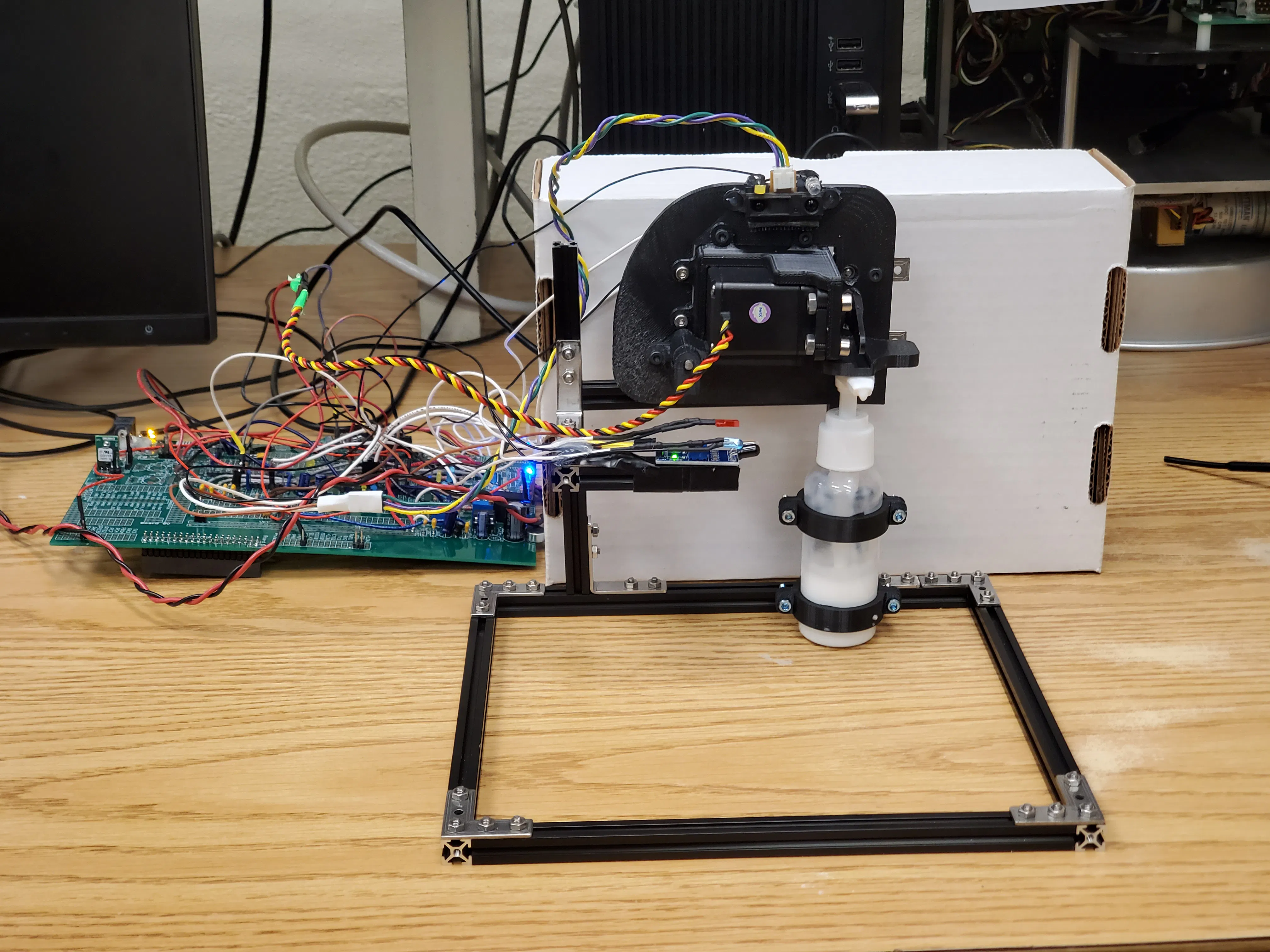### Schematic3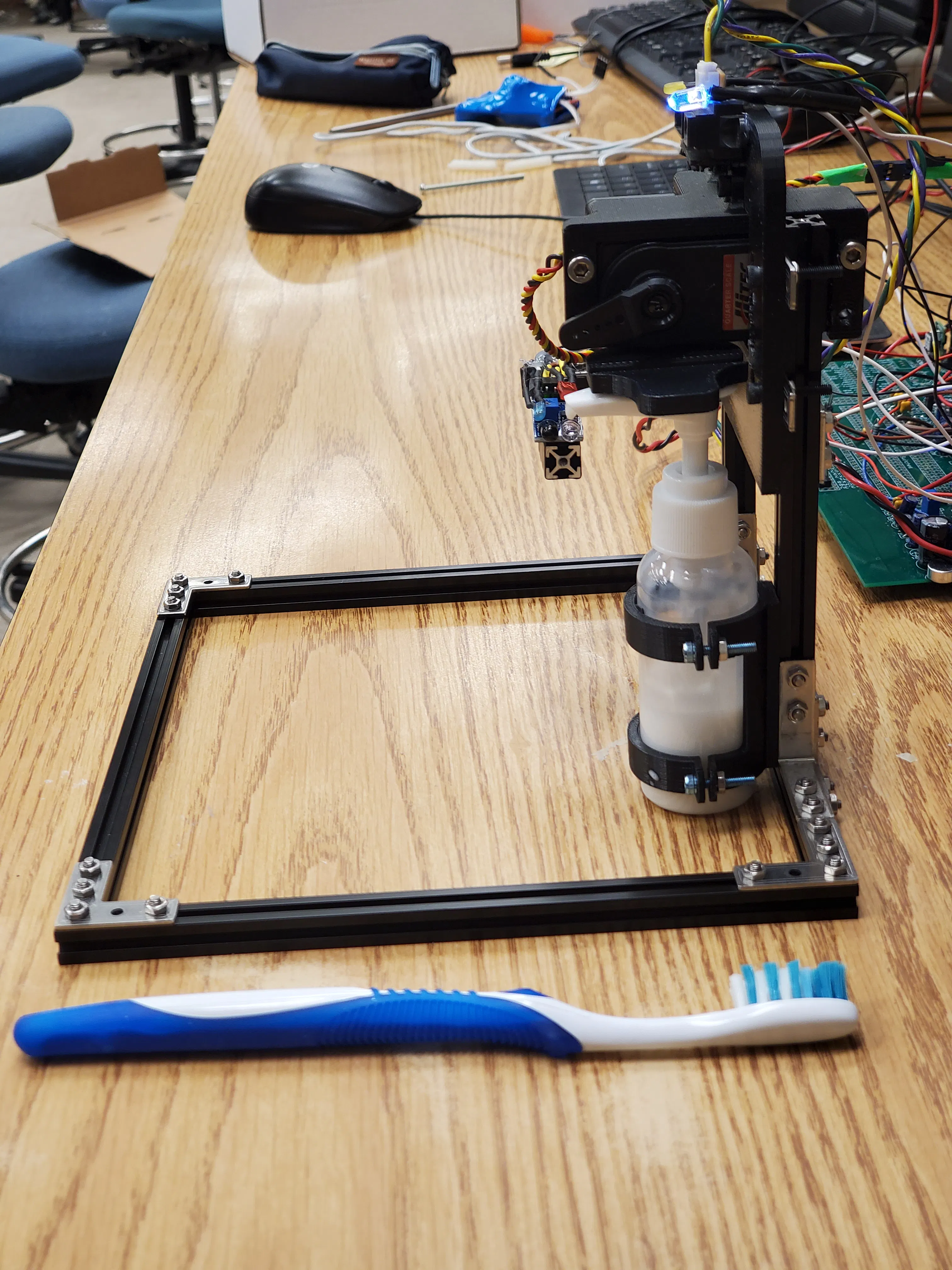### Schematic4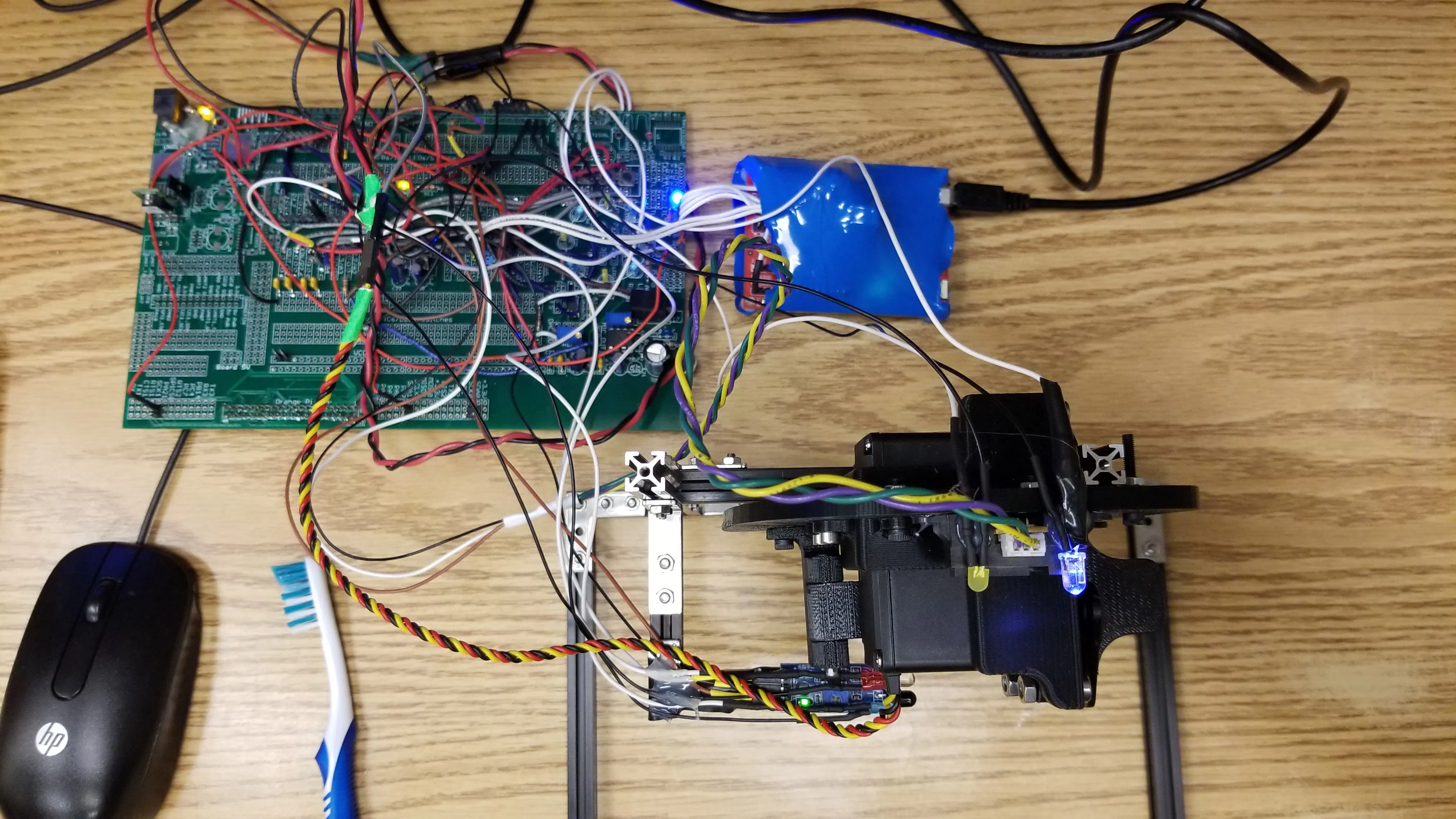## Code

### ToothpasteDispenser_operatingCode

C/C++
```/******************************************************************************
MSP430F2272 Project Creator 4.0

ME 461 - S. R. Platt
Fall 2010

Updated for CCSv4.2 Rick Rekoske 8/10/2011

Written by: Steve Keres
College of Engineering Control Systems Lab
University of Illinois at Urbana-Champaign
*******************************************************************************/

#include "msp430x22x2.h"
#include "UART.h"

// ServoMotor COntrol
#define SERVO_LOW   2000
#define SERVO_HIGH  4500
#define SERVO_INCR  100
int servo_trig1 = 0;
int servo_trig2 = 0;

// Brushing Parameters
#define T_BRUSHING  3000
#define T_GARGLLING 3000
#define T_HOLD1     50
#define T_HOLD2     50
#define T_HOLD_FINISH     200
#define THRS_DIST   100

//MSP430 Operation Counter / flags
char newprint = 0;
unsigned int timecnt = 0;
unsigned int fastcnt = 0;
char sw_state = 0;
char ledcnt =0;
int pwmH = 0;
char print_state(void);
int incr = SERVO_INCR;

//Communication
int RXData = {0,0,0,0,0,0,0,0};
int TXData = {0,0,0,0,0,0,0,0};
long rx1 = 0;
long rx2 = 0;
char rxcnt = 0;
char txcnt = 0;

int state = 1;
unsigned long fastcnt_01 = 0;
int tCount1 = 0;
int tCount2 = 0;
int irDist = 0;

char flagSQZ = 0;
char flagBrush = 0;
char flagDisp = 0;  // {1,2,3,4,5} message to Orange Pi

void main(void) {
WDTCTL = WDTPW + WDTHOLD; // Stop WDT
if (CALBC1_16MHZ ==0xFF || CALDCO_16MHZ == 0xFF) while(1);

DCOCTL  = CALDCO_16MHZ; // Set uC to run at approximately 16 Mhz
BCSCTL1 = CALBC1_16MHZ;

//P1IN          Port 1 register used to read Port 1 pins setup as Inputs // LED code
P1SEL &= ~0xFF; // Set all Port 1 pins to Port I/O function
P1SEL |= 0x04;
P1DIR |=0x04;
P1REN &= ~0xFF; // Disable internal resistor for all Port 1 pins

//P2 (using 4567, 45 pullup, 67 pulldown)
P2SEL &= ~0xFF; // all zeros for GPIO
P2IE  |= 0xF0; //set 1111 0000
P2DIR &= ~0xF0; // 0000 1111 (LOW: input)
P2REN |= 0xF0; // 1111 0000 (HIGH: Enable)
P2OUT &= ~0xFF;// 1100 0000 (HIGH: Pull up)
P2OUT |= 0xC0; // 1100 0000 (HIGH: Pull up)
P2IES |= 0xC0; // 1: rising 0: falling  / 45 pins are pull down
P2IFG &= ~0xFF;

//P3 settings
P3SEL |= 0x06;
UCB0CTL1 = UCSWRST + UCSSEL_2;
UCB0CTL0 = UCMODE_3 + UCSYNC;
UCB0I2COA = 0x25;
UCB0CTL1 &= ~UCSWRST;
IE2 |= UCB0RXIE;
IFG2 &= ~0xFF;

//P4 config.
P4SEL &= ~0xFF; // Set all Port 1 pins to Port I/O function
P4SEL |= 0x10;
P4DIR |= 0x10;
P4REN &= ~0xFF; // Disable internal resistor for all Port 1 pins

// Timer A Config
TACCTL0 = CCIE;              // Enable Timer A interrupt
TACCR0  = 32000;             // period = 2ms
TACTL   = TASSEL_2 + MC_1;   // source SMCLK, up mode

// Timer B Config
TBCCTL0 = 0;                //
TBCCTL1 = OUTMOD_7+CLLD_1;  //
TBCCR0  = 16000;             // period = 1ms
TBCCR1  = SERVO_HIGH;
TBCTL   = TBSSEL_2 + ID_3 + MC_1 ;   // source SMCLK, up mode

Init_UART(115200, 1);   // Initialize UART for 9600 baud serial communication

_BIS_SR(GIE);       // Enable global interrupt

while(1) {
if (newprint==1)  {
UART_printf("%d \t %d \t %d \t %d \t %d\n\r", state, flagBrush, readADC, tCount1, tCount2);
newprint = 0;
}
}
}

// Timer A0 interrupt service routine
#pragma vector=TIMERA0_VECTOR
__interrupt void Timer_A (void)
{
flagBrush = ((~P2IN & 0x10)>>4);
fastcnt++;
if (fastcnt == 50) { //every 0.1s
newprint = 1;
fastcnt = 0;

if ((state ==1 ) && (irDist < THRS_DIST)) { //object is far away
flagDisp = 1; //"IDLE"
tCount1 = 0;
}
else if ((state ==1 ) && (irDist >= THRS_DIST)) { //object is close
flagDisp = 1; //"Welcome"
tCount1++;
if (tCount1 > T_HOLD1) {
tCount1 = 0;
state = 2;
flagDisp = 2; // "It is time to brush your teeth, place your toothbrush under the dispenser"
}
}
else if ((state == 2) && (flagBrush == 0)) {
flagDisp = 2;
tCount2 = 0;
}
else if ((state == 2) && (flagBrush == 1)) {
flagDisp = 3; // "Recognizing your toothbrush"
tCount2++;
if(tCount2 > T_HOLD2) {
//servo motor control
TBCCR1 += incr;
if (TBCCR1 >= SERVO_HIGH && servo_trig1 == 0 && servo_trig2 == 0){
incr = -SERVO_INCR;
servo_trig1 = 1;
}
if (TBCCR1 <= SERVO_LOW && servo_trig1 == 1) {
tCount2 = 0;
state = 3;
flagSQZ = 1;
servo_trig1 = 0;
flagDisp = 4; // "Start toothbrushing. If you don't, you will lose your research funding"
}
if (TBCCR1 <= SERVO_LOW && servo_trig2 == 0 && servo_trig1 == 0) {
incr = SERVO_INCR;
servo_trig2 = 1;
}
if (TBCCR1 >= SERVO_HIGH && servo_trig2 == 1) {
tCount2 = 0;
state = 3;
flagSQZ = 1;
servo_trig2 = 0;
flagDisp = 4; // "Start toothbrushing. If you don't, you will lose your research funding"
}
}
}
else if (state == 3) {
tCount2++;
if (tCount2 > T_BRUSHING) {
flagDisp = 5; // "Now, gargle your mouth"
state = 4;
tCount2 = 0;
}
}
else if (state == 4) {
tCount2++;
if (tCount2 > T_GARGLLING) {
flagDisp = 6; //"FInished"
tCount2 = 0;
// delay(T_HOLD_FINISH);
state = 1;
}
}
}
}

// ADC 10 ISR - Called when a sequence of conversions (A7-A0) have completed
}

// USCI Receive ISR - Called when shift register has been transferred to RXBUF
// Indicates completion of TX/RX operation
#pragma vector=USCIAB0TX_VECTOR
__interrupt void USCI0TX_ISR(void) {

if((IFG2&UCA0TXIFG) && (IE2&UCA0TXIE)) {        // USCI_A0 requested TX interrupt
if(printf_flag) {
if (currentindex == txcount) {
senddone = 1;
printf_flag = 0;
IFG2 &= ~UCA0TXIFG;
} else {
UCA0TXBUF = printbuff[currentindex];
currentindex++;
}
} else if(UART_flag) {
if(!donesending) {
UCA0TXBUF = txbuff[txindex];
if(txbuff[txindex] == 255) {
donesending = 1;
txindex = 0;
} else {
txindex++;
}
}
}
IFG2 &= ~UCA0TXIFG;
}

if((IFG2&UCB0RXIFG) && (IE2&UCB0RXIE)) {    // USCI_B0 RX interrupt occurs here for I2C
RXData[rxcnt] = UCB0RXBUF;
rxcnt++;

if (rxcnt > 7){
TXData = (unsigned char)(state); //msb
TXData = (unsigned char)(flagDisp);
TXData = (unsigned char)(flagBrush);

TXData = (unsigned char)(0); //msb
TXData = (unsigned char)(0);
TXData = (unsigned char)(0);
TXData = (unsigned char)(0);  //lsb

rxcnt = 0;
P1OUT ^= 0x01;
//newprint = 1;
// rx1 = pixel cnt, rx2 = centroid column
rx1 = (RXData) + ((long)RXData << 8) + ((long)RXData << 16) + ((long)RXData << 24);
rx2 = (RXData) + ((long)RXData << 8) + ((long)RXData << 16) + ((long)RXData << 24);

// TBCCR1 = rx1;
IE2 &= ~UCB0RXIE;
IE2 |= UCB0TXIE;
}
} else if ((IFG2&UCB0TXIFG) && (IE2&UCB0TXIE)) { // USCI_B0 TX interrupt
// put your TX code here.
UCB0TXBUF=  TXData[txcnt];
txcnt++;
if (txcnt >7){
txcnt = 0;
P1OUT ^= 0x02;
IE2 &= ~UCB0TXIE;
IE2 |= UCB0RXIE;
}
}
}

#pragma vector=PORT2_VECTOR
__interrupt void Port_2(void)
{
if ((P2IFG & 0x10) == 0x10){
flagBrush = 1;
P2IFG &= ~0x10;
}
if ((P2IFG & 0x20) == 0x20){
P2IFG &= ~0x20;
}
if ((P2IFG & 0x40) == 0x40){
P2IFG &= ~0x40;
}
if ((P2IFG & 0x80) == 0x80){
P2IFG &= ~0x80;
}

}
```

## Credits

### Jaejun Park

1 project • 0 followers

### Youngwoo Sim

1 project • 0 followers